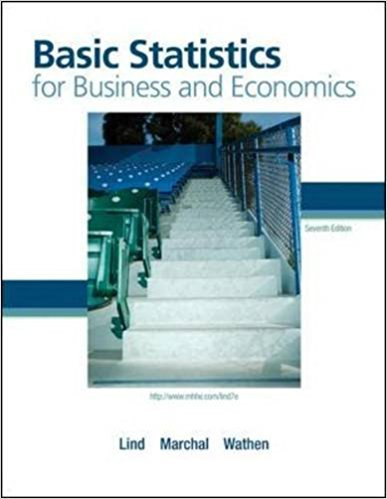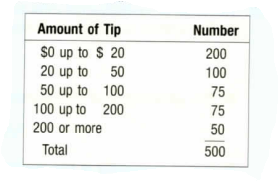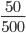×
Get Full Access to Basic Statistics For Business And Economics - 7 Edition - Chapter 5 - Problem 44e
Get Full Access to Basic Statistics For Business And Economics - 7 Edition - Chapter 5 - Problem 44e

×

Berdine’s Chicken Factory has several stores in the HiltonISBN: 9780077384470 64

Solution for problem 44E Chapter 5

Basic Statistics for Business and Economics | 7th Edition

• Textbook Solutions
• 2901 Step-by-step solutions solved by professors and subject experts
• Get 24/7 help from StudySoup virtual teaching assistantsBasic Statistics for Business and Economics | 7th Edition

4 5 1 249 Reviews
22
5
Problem 44E

Berdine's Chicken Factory has several stores in the Hilton Head, South Carolina, area. When interviewing applicants for server positions, the owner would like to include information on the amount of tip a server can expect to earn per check (or bill). A study of 500 recent checks indicated the server earned the following amounts in tips per 8-hour shift.a. What is the probability of a tip of $200 or more? b. Are the categories "$0 up to $20”, “$20 up to $50," and so on considered mutually exclusive? c. If the probabilities associated with each outcome were totaled, what would that total be? d. What is the probability of a tip of up to$50 ?

e. What is the probability of a tip of less than $200 ? Step-by-Step Solution: Step 1 of 5 Given a study of 500 recent checks indicated the server earned. Then the table is given below.  Amount of Tip Number$0 up to $20 200$20 up to $50 100$50 up to $100 75$100 up to $200 75$200 or more 50 Total 500

Our goal is:

a). We need to find the probability of a tip of $200 or more. b). We need to find are the categories mutually exclusive. c). We need to find the total probability. d). We need to find the probability of a tip of up to$50.

e). We need to find the probability of less than $200. a). Now we have to find the probability of a tip of$200 or more.

From the above table the amount tip $200 or more = 50 (Number) and The total number of amount tip is 500. Then the probability of tip of$200 or more is

P (tip of $200 or more) =P (tip of$200 or more) = 0.1

Therefore, the probability of tip of \$200 or more is 0.1.

____________________________________________________________________________

Step 2 of 5

Step 3 of 5

ISBN: 9780077384470

Unlock Textbook Solution